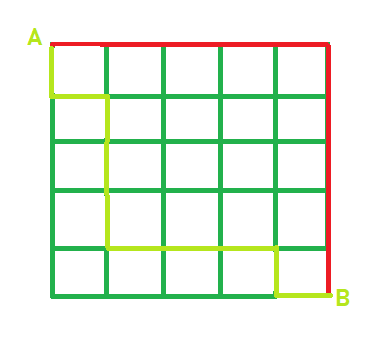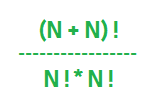GFG App
Open AppBrowser
Continue

# Number of ways to go from one point to another in a grid

Given the NxN grid of horizontal and vertical roads. The task is to find out the number of ways that the person can go from point A to point B using the shortest possible path.
Note: A and B point are fixed i.e A is at top left corner and B at bottom right corner as shown in the below image.In the above image, the path shown in the red and light green colour are the two possible paths to reach from point A to point B.
Examples:

```Input: N = 3
Output: Ways = 20

Input: N = 4
Output: Ways = 70```

Formula:
Let the grid be N x N, number of ways can be written as.How does above formula work?
Let consider the example of the 5×5 grid as shown above. In order to go from point A to point B in the 5×5 grid, We have to take 5 horizontal steps and 5 vertical steps. Each path will be an arrangement of 10 steps out of which 5 steps are identical of one kind and other 5 steps are identical of a second kind. Therefore
No. of ways = 10! / (5! * 5!) i.e 252 ways.

## C++

 `// C++ implementation of above approach` `#include ` `using` `namespace` `std;`   `// function that will` `// calculate the factorial` `long` `factorial(``int` `N)` `{` `    ``int` `result = 1;` `    ``while` `(N > 0) {` `        ``result = result * N;` `        ``N--;` `    ``}` `    ``return` `result;` `}`   `long` `countWays(``int` `N)` `{` `    ``long` `total = factorial(N + N);` `    ``long` `total1 = factorial(N);` `    ``return` `(total / total1) / total1;` `}`   `// Driver code` `int` `main()` `{` `    ``int` `N = 5;` `    ``cout << ``"Ways = "` `<< countWays(N);` `    ``return` `0;` `}`

## Java

 `// Java implementation of above approach` `class` `GfG {`   `    ``// function that will` `    ``// calculate the factorial` `    ``static` `long` `factorial(``int` `N)` `    ``{` `        ``int` `result = ``1``;` `        ``while` `(N > ``0``) {` `            ``result = result * N;` `            ``N--;` `        ``}` `        ``return` `result;` `    ``}`   `    ``static` `long` `countWays(``int` `N)` `    ``{` `        ``long` `total = factorial(N + N);` `        ``long` `total1 = factorial(N);` `        ``return` `(total / total1) / total1;` `    ``}`   `    ``// Driver code` `    ``public` `static` `void` `main(String[] args)` `    ``{` `        ``int` `N = ``5``;` `        ``System.out.println(``"Ways = "` `+ countWays(N));` `    ``}` `}`

## Python3

 `# Python3 implementation of above approach `   `# function that will calculate the factorial ` `def` `factorial(N) : ` `    `  `    ``result ``=` `1``; ` `    `  `    ``while` `(N > ``0``) :` `        `  `        ``result ``=` `result ``*` `N; ` `        ``N ``-``=` `1``; ` `    `  `    ``return` `result; `   `def` `countWays(N) : `   `    ``total ``=` `factorial(N ``+` `N); ` `    ``total1 ``=` `factorial(N); ` `    `  `    ``return` `(total ``/``/` `total1) ``/``/` `total1; `   `# Driver code ` `if` `__name__ ``=``=` `"__main__"` `: `   `    ``N ``=` `5``; ` `    `  `    ``print``(``"Ways ="``, countWays(N)); `   `# This code is contributed by Ryuga`

## C#

 `// C# implementation of above approach ` `using` `System; ` `class` `GfG ` `{ `   `    ``// function that will ` `    ``// calculate the factorial ` `    ``static` `long` `factorial(``int` `N) ` `    ``{ ` `        ``int` `result = 1; ` `        ``while` `(N > 0) ` `        ``{ ` `            ``result = result * N; ` `            ``N--; ` `        ``} ` `        ``return` `result; ` `    ``} `   `    ``static` `long` `countWays(``int` `N) ` `    ``{ ` `        ``long` `total = factorial(N + N); ` `        ``long` `total1 = factorial(N); ` `        ``return` `(total / total1) / total1; ` `    ``} `   `    ``// Driver code ` `    ``public` `static` `void` `Main(String []args) ` `    ``{ ` `        ``int` `N = 5; ` `        ``Console.WriteLine(``"Ways = "` `+ countWays(N)); ` `    ``} ` `} `   `// This code is contributed by Arnab Kundu`

## PHP

 ` 0) ` `    ``{` `        ``\$result` `= ``\$result` `* ``\$N``;` `        ``\$N``--;` `    ``}` `    ``return` `\$result``;` `}`   `function` `countWays(``\$N``)` `{` `    ``\$total` `= factorial(``\$N` `+ ``\$N``);` `    ``\$total1` `= factorial(``\$N``);` `    ``return` `(``\$total` `/ ``\$total1``) / ``\$total1``;` `}`   `// Driver code` `\$N` `= 5;` `echo` `"Ways = "``, countWays(``\$N``);` `    `  `// This code is contributed by ajit` `?>`

## Javascript

 ``

Output:

`Ways = 252`

My Personal Notes arrow_drop_up